Sewing

Beth's mother can sew 235 pairs of short pants in 6 days while Lourdes can sew 187 pairs in 8 days. How many more pairs of short pants can Beth's mother sew?

Result

x =  15.917

Solution:Leave us a comment of this math problem and its solution (i.e. if it is still somewhat unclear...):Be the first to comment!To solve this verbal math problem are needed these knowledge from mathematics:

Need help calculate sum, simplify or multiply fractions? Try our fraction calculator.

Next similar math problems:

1. Tractors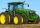6 tractors started plowing the field that them together take 12 days. Agronomist hesitates to A) after 2 days to withdraw 2 tractors, or B) after 3 days to withdraw 3 tractors. Help him which of these two cases will be plowing done sooner.
2. Classroom 4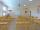In a class of 36 pupils, 2/3 are girls. How much it is in a class girls and boys?
3. Hectoliters of waterThe pool has a total of 126 hectoliters of water. The first pump draws 2.1 liters of water per second. A second pump pumps 3.5 liters of water per second. How long will it take both pumps to drain four-fifths of the water at the same time?
4. Watching TVOne evening 2/3 students watch TV. Of those students, 3/8 watched a reality show. Of the students that watched the show, 1/4 of them recorded it. What fraction of the students watched and recorded reality tv.
5. Summerjobs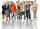The agency give summerjobs for 2352 students in 2018. The eighth was high-school students, the rest was undergraduates. How many undergraduates works via agency in 2018?
6. Soup from canteen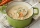For how many people is 90 liters of soup enough if we assume 3/8 liter of soup per person in the canteen?
7. DivideDivide the number 72 in the ratio 7: 2 and calculate the ratio of the numbers found in this order and write down as decimal.
8. Percent calculationCalculate 61% if 85% is 38.
9. Find the 11Find the quotient of 229.12 and 12.32
10. Quotient 3If the quotient of 8/13 and 2 is subtracted from the product of 1 3/4 and 8/21, what is the difference?
11. TogetherGrandfather, father, and son are carpenters. They decided to make a wardrobe. Father produces it for 12 hours, his son for 15 hours, and grandfather for 10 hours. How long will they make it together?
12. Balls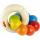The boys changed stamps, beads and balls. For 9 balls is 3 stamps, 2 balls is 44 stamps. How many beads is for 1 ball?
13. EmployeesOf all 360 employees, there are 11/12 women. How many men work in a company?
14. The squareThe square root of 25 times the square root of 81 is what number?
15. Women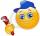In an company of 1050 employees are 2/3 women. How many women work in the company?
16. InqualitySolve inequality: 3x + 6 > 14
17. Percentages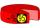Expressed as a percentage: